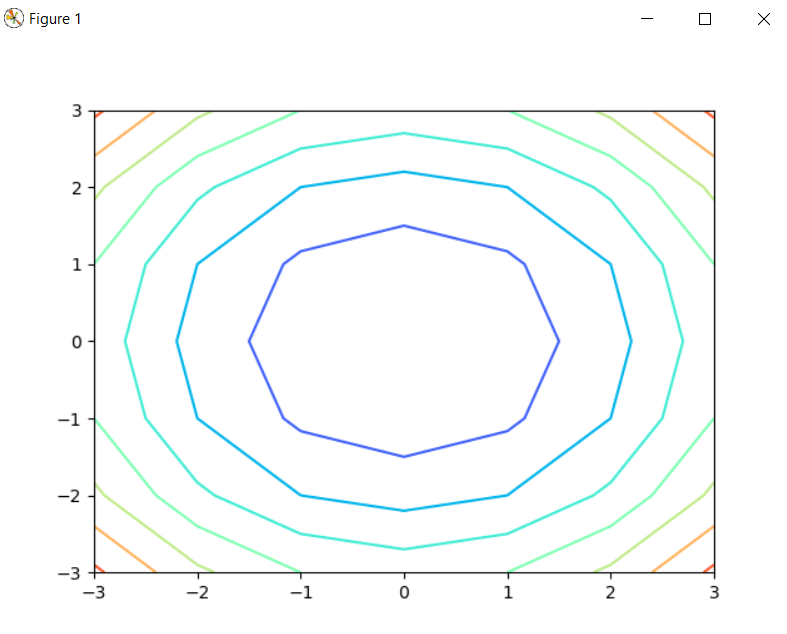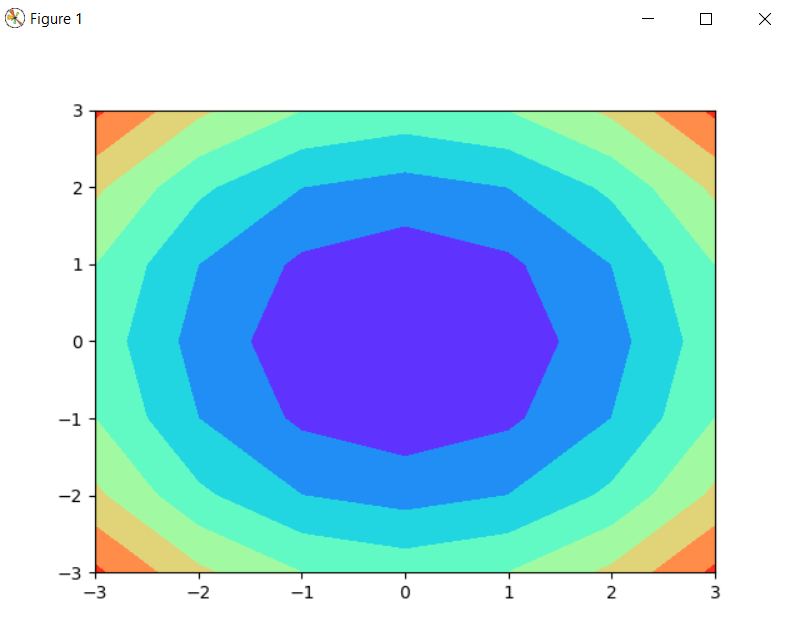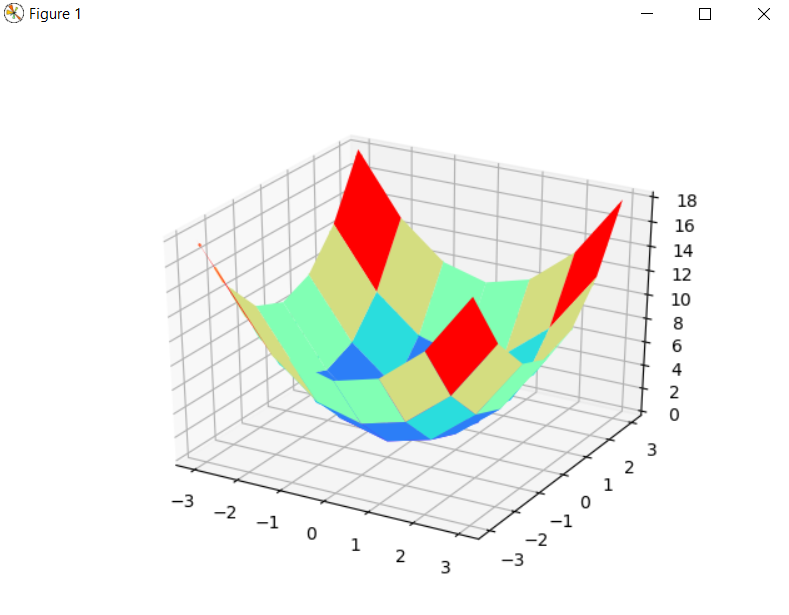# Contourf plot in matplotlib using Python

In this article, we will learn about the concept of the contourf plot in matplotlib using Python. contourf function uses the current colormaps to fill the spaces between the levels in the plot. The main use of contourf function is to fill color between the lowest level and the level above it, and the first color in the contourf function fills the space with provided color between the lowest level and the level above it. The last color value in the contourf function represents the Z-axis values that are greater than the highest level present in the plot.

## Contourf plot in matplotlib using Python

For plotting the contourf plot we have to plot the contour plot first, as contourf function fills the spaces between the levels of contour in the plot, then we have to plot the contour plot for which we have to use the meshgrid function which creates a two-dimensional grid from one-dimensional array with the help of numpy.

Meshgrid: It always returns the two-dimensional array which represents the x and y coordinates of all the points. Let us understand with one example:

### Plotting of Contour plot(2-D)

```import matplotlib.pyplot as plt
import numpy as np

A=np.array([-3,-2,-1,0,1,2,3])
B=A
A,B=np.meshgrid(A,B)
fig = plt.figure()

plt.contour(A,B,A**2+B**2)
plt.show()```

OutputIn this example, we first imported the required library numpy and matplotlib and then created a numpy array and stored it in the variable named as A and then established the relation between A and B. After that, we used the meshgrid function and passed A and B inside it, then we plotted the contour by passing 3 arguments inside it, A, B, A**2+B**2

### Plotting of Contourf plot(2-D)

```import matplotlib.pyplot as plt
import numpy as np

A=np.array([-3,-2,-1,0,1,2,3])
B=A
A,B=np.meshgrid(A,B)
fig = plt.figure()

plt.contour(A,B,A**2+B**2,cmap="rainbow")
plt.show()```

OutputThe explanation for this example is as same as above, the only difference is that we used the contourf function instead of contour function. The contourf function will only fill the color between the lowest level and the level above it.

### Plotting of Contour plot(3-D)

```from mpl_toolkits.mplot3d import Axes3D
import matplotlib.pyplot as plt
import numpy as np

a=np.array([-3,-2,-1,0,1,2,3])
b=a
a,b=np.meshgrid(a,b)

fig = plt.figure()
axes = fig.gca(projection="3d")
axes.plot_surface(a,b,a**2+b**2,cmap="rainbow")

plt.contour(a,b,a**2+b**2,cmap="rainbow")
plt.show()

```

OutputIn the above example, the contour plot is in three-dimensional, the only change we have done in this example from the previous example is that we imported an extra module Axes3D from mpl_toolkits.mplot3d for three-dimensional plotting.

### Plotting of Contourf plot(3-D)

```from mpl_toolkits.mplot3d import Axes3D
import matplotlib.pyplot as plt
import numpy as np

a=np.array([-3,-2,-1,0,1,2,3])
b=a
a,b=np.meshgrid(a,b)

fig = plt.figure()
axes = fig.gca(projection="3d")
axes.plot_surface(a,b,a**2+b**2,cmap="rainbow")

plt.contourf(a,b,a**2+b**2,cmap="rainbow")
plt.show()

```

Output